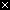# Image-2 Code and Images

In this section, we'll look at simple code to load and manipulate a digital image. Here we'll just manipulate one pixel at a time. In the next section, scaling up to operate on thousands of pixels at a time.

## x.png

• x.png - a tiny example image
• 10 pixels wide by 10 pixels high
• PNG is an image file format, like JPG

The image "x.png" is very simple - it's a very small black square with a white "x" at its center. Here is x.png:PNG (Portable Network Graphics) is a format to store an image in a computer file, like JPG and GIF.

## x.png Code Example

• Three line program
• First line stores image named "x.png" into variable named "image":
• `image = new SimpleImage("x.png");`
• `image.setZoom(20);` -- set zoom option to 20 (10, 20, ... whatever)
• `print(image);` -- print out image as usual
• Zoom: each pixel shown 20x size here

Our first image code example loads the x.png image into a variable and prints it. Run the code to see what it does. Try changing the `image.setZoom(20)` to use the number 10 or 30 instead.

image2-1

## pixel.setRed(255) Example

• Now add some pixel-manipulation lines
• First line gets pixel (0, 0) and stores it in a variable named "pixel":
• `pixel = image.getPixel(0, 0);`
• `pixel.setRed(255);` -- set red value of that pixel
• Try setRed() with different values in the range 0..255

image2-2

## Pixel Set Red/Green/Blue Functions

• `pixel.setRed(number);` -- set the red value of the pixel to be the given number (0..255)
• `pixel.setGreen(number);` -- set the green value
• `pixel.setBlue(number);` -- set the blue value

## noun.verb() Pattern

• Computer code has a widely used patterns
• One, we're seeing here is the noun.verb() pattern
• The noun is the thing we want to operate on (e.g. a pixel)
• The verb (aka "function") operates on that noun
• e.g. the functions setRed and setZoom
• Getting used to this pattern, our code will look less weird

## Aside: Image Functions Reference

For later reference, there is a separate image functions reference page which lists in one place all the image functions such as `pixel.setRed(number)` we are using here.

## Experiments On Pixels (0, 0) and (1, 0)

To see how `image.getPixel(x, y)` and `pixel.setRed(number)` etc. work, we'll try the experiments listed below (use the "show" button to see the solution code).

image2-3

Demo the first few, ask class to do the rest.

Example ProblemsSolution
Set pixel (0, 0) to be green.
Set pixel (0, 0) to be yellow.
Set pixel (1, 0) to be yellow. Where is that pixel?
Set pixel (0, 0) to white.
What is the x,y of the pixel below the origin? Set that pixel to be white.

## Multiple Pixels - Red Diagonal Problem

Change multiple pixels. Technique: assign "pixel" variable with different x,y to access different pixels. This code changes the origin pixel and the pixel to its right to be red:

image2-4

Example ProblemsSolution
Set origin to be green, pixel below it red. (You Do It)
The pixel at (2, 2) is white. Set it to be red. Add print(pixel); lines to illuminate what's going on. (Demo, a bit tricky)
Red diagonal problem: Set 3 pixels to pure red, origin, below it to the right, and below that to the right. (You Try It)